## Some results from group theory

Definition 4   Let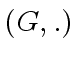be a (multiplicative) group with neutral element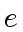. A nonempty subset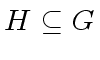is a subgroup of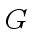if the following three statements hold
•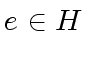,
• for every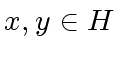we have,
• for every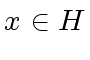the inverse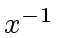of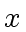belongs to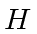.

Theorem 3   For every subgroupof the additive abelian group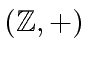there exists an element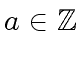such thatis the set of the multiples of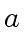, that is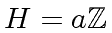.

Theorem 4   Letbe a multiplicative group with neutral element. Let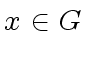an element and gr() the subgroup ofconsisting of all powers of(including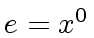andthe inverse of). Let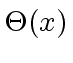be the order of gr(), that is the cardinality of gr(). Then two cases arise
• either gr() is infinite and then the powers ofare pairwise different and thusis isomorphic to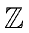.
• or gr() is finite and we have the following properties
1.is the smallest integer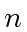such that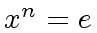,
2.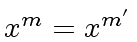iff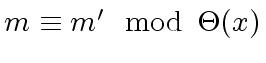,
3.is isomorphic to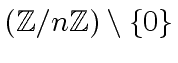where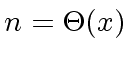,
4.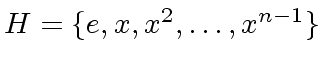where.

Theorem 5 (Lagrange)   For every subgroupof the finite group, the order (that is the cardinality) ofdivides that of.

Marc Moreno Maza
2008-01-07